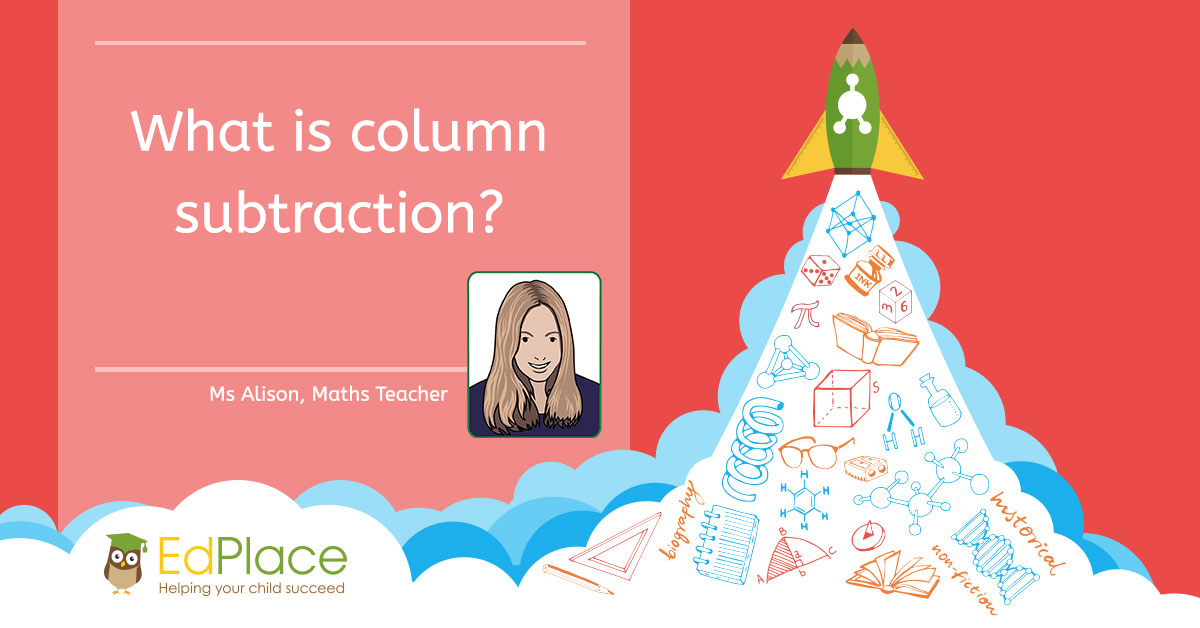What is column subtraction?

Subtraction is simply taking away one number from another. When the numbers are easy, we can subtract them in our heads. However, when the numbers get a little tricker, that's when column subtraction can be an efficient and accurate method. That being said, it only works properly if you understand place value first, otherwise, it’s all too easy to accidentally jumble up the numbers!

How to use column subtraction without having to borrow.

Let's use the column subtraction method to work out 55 - 34.

1. Set out the numbers like this;

 Tens Units 5 5 - 3 4

Always place the biggest number in the top row.

Make sure that even if you don’t label it, you have both a 'tens' column and a 'units' column. Line up the tens directly underneath each other and do the same for the units.

1. Start with the units (or the column furthest to the right if you have decimals).

Always subtract the bottom number(s) from the top even if the top number looks smaller (more on that later).

 Tens Units 5 5 - 3 4 1

5 subtract 4 is 1.

1. Repeat with the tens (and then the hundreds, thousands and so on, if you have any).

 Tens Units 5 5 - 3 4 2 1

5 subtract 3 is 2.

(Actually, this is 50-30=20 but placed within the tens column we don’t need to worry about using the zero as a placeholder).

The answer, 21 is now displayed underneath.

How to use column subtraction with borrowing

If the top digit in any of the columns is smaller than the bottom digit, then we have to borrow.

Tip - You cannot just swap the numbers over and subtract the top from the bottom. Lots of people try this, but it won’t give you the right answer!

Let's work out 132 - 64.

1. Set out the numbers like this;

 Hundreds Tens Units 1 3 2 - 6 4

Make sure that even if you don’t label them, you have a 'hundreds' column, 'tens' column and a 'units' column. Line up the tens directly underneath each other and do the same for the units.

Tip - If the number you are subtracting has less digits (as in our example), make sure that the tens are always placed below the tens, units below units. Leave a space or put a zero to make sure that you don’t accidentally shift your digits into the wrong columns.

1. Start with the units (or the column furthest to the right if you have decimals).

Always subtract the bottom number(s) from the top even if the top number looks smaller. In this case we need to take 4 away from 2. How?! You may ask.

We do this by borrowing.

There are 3 tens or 30 in 132. We simply ‘borrow’ one of the tens. 30 becomes 20 and then the extra ten is added to the 2 units to make 12.

Now our calculation becomes 12 – 4 = 8.

 Hundreds Tens Units 1 2 3 12 - 6 4 8

1. Repeat with the tens (or the next column to the left).

Once again we will have to borrow because you can’t take away 60 from 20.

We simply ‘borrow’ one hundred, which will leave us with zero in the hundreds column and 120 in the tens column.

Our calculation now becomes 120 – 60 = 60

(As we are calculating in columns we do not need to show the 0 of 120 so it looks like 12 but is actually 120)

 Hundreds Tens Units 1 12 3 12 - 6 4 6 8

The answer, 68 is now displayed underneath.

Worksheets and Practice

We have loads of great worksheets to teach you about using column subtraction. We’ve listed a few of the most relevant here, but browse through our website or search for ‘subtract’ to find more resources.

Year 3 – Practice your subtraction 1 2a 2b 2c 3

Year 3 – Subtracting 2-digit numbers

Year 5 – Add and subtract problems

Year 7 – Practise decimal subtraction

Further Information

If you enjoy using column subtraction and want to give yourself a challenge, why not try some of the puzzles and problems set by the NRich team from University of Cambridge?

Here are a selection of puzzles and problems linked to using column subtraction to really get you thinking!

https://nrich.maths.org/8955

https://nrich.maths.org/public/search.php?search=column+subtraction+ks2

Keep learning with EdPlace! More activities this way…AUTHOR, MS ALISON – ENGLISH TEACHER